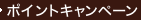•### Essential Calculus

• ただいまウェブストアではご注文を受け付けておりません。 ⇒古書を探す
• 商品コード 9780495108603

### Full Description

This book is a response to those instructors who feel that calculus textbooks are too big. In writing the book James Stewart asked himself: What is essential for a three-semester calculus course for scientists and engineers? Stewart's ESSENTIAL CALCULUS offers a concise approach to teaching calculus that focuses on major concepts and supports those concepts with precise definitions, patient explanations, and carefully graded problems. Essential Calculus is only 850 pages-two-thirds the size of Stewart's other calculus texts (CALCULUS, Fifth Edition and CALCULUS, EARLY TRANSCENDENTALS, Fifth Edition)-and yet it contains almost all of the same topics. The author achieved this relative brevity mainly by condensing the exposition and by putting some of the features on the website, www.StewartCalculus.com. Despite the reduced size of the book, there is still a modern flavor: Conceptual understanding and technology are not neglected, though they are not as prominent as in Stewart's other books. ESSENTIAL CALCULUS has been written with the same attention to detail, eye for innovation, and meticulous accuracy that have made Stewart's textbooks the best-selling calculus texts in the world.

### Contents

1. FUNCTIONS AND LIMITS. Functions and Their Representations. A Catalog of Essential Functions. The Limit of a Function. Calculating Limits. Continuity. Limits Involving Infinity. Review. 2. DERIVATIVES. Derivatives and Rates of Change. The Derivative as A Function. Basic Differentiation Formulas. The Product and Quotient Rules. The Chain Rule. Implicit Differentiation. Related Rates. Linear Approximations and Differentials. Mean Value Theorem. Derivatives and the Shapes of Graphs. Curve Sketching. Optimization Problems. Newton's Method. Antiderivatives. Review. 4. INTEGRALS. Areas and Distances. The Definite Integral. Evaluating Definite Integrals. The Fundamental Theorem of Calculus . The Substitution Rule. Review. 5. INVERSE FUNCTIONS. Inverse Functions. The Natural Logarithmic Function. The Natural Exponential Function. General Logarithmic and Exponential Functions. Exponential Growth and Decay. Inverse Trigonometric Functions. Hyperbolic Functions. Indeterminate Forms and l'Hospital's Rule. Integrals and Substitutions. Partial Fractions. Integration with Tables and Computer Algebra Systems. Approximate Integration. Improper Integrals. Volumes by Cylindrical Shells. Arc Length. Applications to Physics and Engineering. Differential Equations. Review. 8. SERIES. Sequences. Series. The Integral and Comparison Tests. Other Convergence Tests. Power Series. Representing Functions as Power Series. Taylor and Maclaurin Series. Applications of Taylor Polynomials. Review. 9. PARAMETRIC EQUATIONS AND POLAR COORDINATES. Parametric Curves. Calculus with Parametric Curves. Polar Coordinates. Areas and Lengths in Polar Coordinates. Conic Sections in Polar Coordinate Systems. Vectors. The Dot Product. The Cross Product. Equations of Lines and Planes. Cylinders and Quadric Surfaces. Vector Functions and Space Curves. Arc Length and Curvature. Motion in Space: Velocity and Acceleration. Review. 11. PARTIAL DERIVATIVES. Functions of Several Variables. Limits and Continuity. Partial Derivatives. Tangent Planes and Linear Approximations. The Chain Rule. Directional Derivatives and the Gradient Vector. Maximum and Minimum Values. Lagrange Multipliers. Review. 12. MULTIPLE INTEGRALS. Double Integrals over Rectangles. Double Integrals over General Regions. Double Integrals in Polar Coordinates. Applications of Double Integrals. Triple Integrals. Triple Integrals in Cylindrical Coordinates. Triple Integrals in Spherical Coordinates. Change of Variables in Multiple Integrals. Review. 13. VECTOR CALCULUS. Vector Fields. Line Integrals. The Fundamental Theorem for Line Integrals. Green's Theorem. Curl and Divergence. Parametric Surfaces and Their Areas. Surface Integrals. Stokes' Theorem. The Divergence Theorem. Review. Appendix A: Trigonometry. Appendix B: Proofs. Appendix C: Sigma Notation.